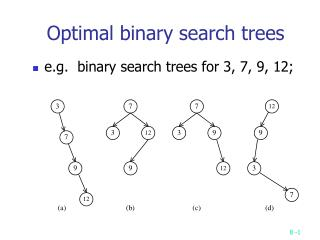DownloadDownload PresentationOptimal binary search trees

# Optimal binary search trees

Télécharger la présentation## Optimal binary search trees

- - - - - - - - - - - - - - - - - - - - - - - - - - - E N D - - - - - - - - - - - - - - - - - - - - - - - - - - -
##### Presentation Transcript

1. Optimal binary search trees • e.g. binary search trees for 3, 7, 9, 12;

2. Optimal binary search trees • n identifiers : a1 <a2 <a3 <…< an Pi, 1in : the probability that ai is searched. Qi, 0in : the probability that x is searched where ai < x < ai+1 (a0=-, an+1=).

3. Identifiers : 4, 5, 8, 10, 11, 12, 14 • Internal node : successful search, Pi • External node : unsuccessful search, Qi • The expected cost of a binary tree: • The level of the root : 1

4. The dynamic programming approach • Let C(i, j) denote the cost of an optimal binary search tree containing ai,…,aj . • The cost of the optimal binary search tree with ak as its root :

5. General formula

6. Computation relationships of subtrees • e.g. n=4 • Time complexity : O(n3) when j-i=m, there are (n-m) C(i, j)’s to compute. Each C(i, j) with j-i=m can be computed in O(m) time.

7. Matrix-chain multiplication • n matrices A1, A2, …, An with size p0  p1, p1  p2, p2  p3, …, pn-1  pn To determine the multiplication order such that # of scalar multiplications is minimized. • To compute Ai  Ai+1, we need pi-1pipi+1 scalar multiplications. e.g. n=4, A1: 3  5, A2: 5  4, A3: 4  2, A4: 2  5 ((A1 A2)  A3)  A4, # of scalar multiplications: 3 * 5 * 4 + 3 * 4 * 2 + 3 * 2 * 5 = 114 (A1 (A2 A3))  A4, # of scalar multiplications: 3 * 5 * 2 + 5 * 4 * 2 + 3 * 2 * 5 = 100 (A1 A2)  (A3 A4), # of scalar multiplications: 3 * 5 * 4 + 3 * 4 * 5 + 4 * 2 * 5 = 160

8. Let m(i, j) denote the minimum cost for computing Ai  Ai+1  … Aj • Computation sequence : • Time complexity : O(n3)